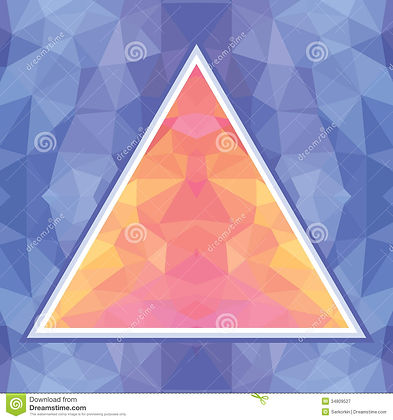# KS3 and GCSE 9-1 Curriculum

## DATES AND SESSIONS# KS3-4 Curriculum

## DATES AND SESSIONSCONCEPTUAL UNDERSTANDING

The principles and practicalities behind conceptual maths tecahing in the classroomTHE NUMBER SYSTEM

Kay Lowdon / Sophie Kardi / Tom Marjoram

Understanding place value and the properties of numbersCALCULATION STRATEGIES

Charlie Dawson

Effective addition, subtraction, multiplication and division strategiesPROPORTIONALITY

Katy Doidge

Understanding fractions, decimals, percentages, ratio and proportionalityPROBABILITY

Sophie Kardi

Effective strategies to teach probability for conceptual understandingTRANSFORMATIONS

Andrew Gemmell

Understanding how geometrical shapes and functions may be transformed

ResourcesGEOMETRICAL REASONING

Craig Stuart

Geometrical problem solving in the new curriculumALGEBRA AND LINEAR EQUATIONS

Charlie Dawson

Introducing algebra and working with the unknown in a linear contextMEASURES

Understanding perimeter, area and volume and their interconnectionsQUADRATICS

Further algebra and working with the unknown in a quadratic contextPYTHAGORAS AND TRIGONOMETRY

Effective strategies to introduce these 9-1 Foundation tier topicsPROBLEM SOLVING

Nicola Coe

Strategies to enable students to construct and solve mathematical problemsEFFECTIVE MATHS TEACHING

5 big ideas that support effective maths teaching in the new curriculum

* Please note that as a result of Covid 19 the remaining sessions will now be delivered online.